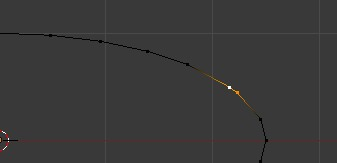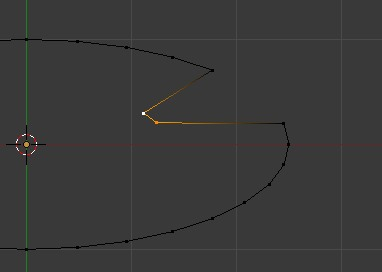# mesh vertex pivot_point

Hi:) i have problem. I have ellipse and i want to scale one of the vertex.I mean pivot is cursor center which is inside ellipse. When i do it “by myself” not by python it work,but when I run script it doesn’t work at all.Also when more than one vertex is selected it doesn’t work too.In fact it works just like “median point” be turned on,But “3D cursor” is turned on.

CODE

import bpy

if bpy.context.mode == ‘EDIT_MESH’:
bpy.ops.object.editmode_toggle()

bpy.context.area.type = ‘VIEW_3D’
############################
space = bpy.context.space_data
space.pivot_point = “CURSOR”

bpy.ops.object.editmode_toggle ()

bpy.ops.transform.resize(value=(0.9, 0.9, 0.9))

#bpy.ops.object.editmode_toggle ()
############################
bpy.context.area.type=“TEXT_EDITOR”
How it works when i do it manual.When i use python it doesn’t work.

When i have 2 vertex active and use pythonI do not have any idea what it work in this way.My question is if anybody has got idea what is wrong?

hi, this works

``````
import bpy
bpy.ops.transform.translate (value=(-0.1, 0.0, 0.0))

``````

not sure if it’s what you need.

Not exactly.Your reply is ok if I want to move only one vertex.if I could scale it in way i would like i will get possibility to change “radius” of the object. for example if selected would be vertex 1 and 2 i could get “radious” bigger (pivot is 3d coursor all the time) and for example vertex deselect all vertex,select vertex 3,4,5 and get “radious” smaller etc.

The main problem is eaven 3d cursor pivot point is selected it scale as if median point was selected. It doesn’t matter if I scale something in object mode or in edit mode.I think that i must use some extra function or something.